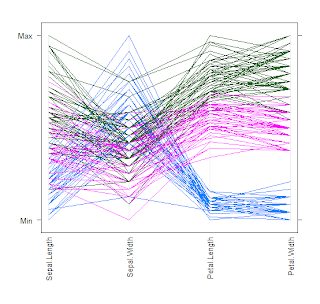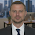## Wednesday, March 11, 2009

### Andrews' Curve And Parallel Coordinate Graph

Unison graph and parallel coordinate graph share similar thought in visualising the difference of multidimensional data, thought the former is much more complicated. Based on iris data, we can see their performance.
• Parallel coordinate graph• Andrews' CurveWe can see that unison graph seems more vivid and powerful.

#----------------------------------------------------------------------
#code of unison graph
x=as.matrix(iris[1:4])
t<-seq(-pi, pi, pi/30) m<-nrow(x); n<-ncol(x) f<-matrix(0, c(m,length(t))) for(i in 1:m){ f[i,]<-x[i,1]/sqrt(2) for( j in 2:n){ if (j%%2==0) f[i,]<-f[i,]+x[i,j]*sin(j/2*t) else f[i,]<-f[i,]+x[i,j]*cos(j%/%2*t) } } plot(c(-pi,pi), c(min(f),max(f)), type="n", main="The Unison graph of Iris", xlab="t", ylab="f(t)") for(i in 1:m) lines(t, f[i,] , col=c("red", "green3", "blue")[unclass(iris\$Species[i])]) legend(x=-3,y=15,c('setosa','versicolor', 'virginica'), lty=1,col=c("red", "green3", "blue"))

1.2.3.4.